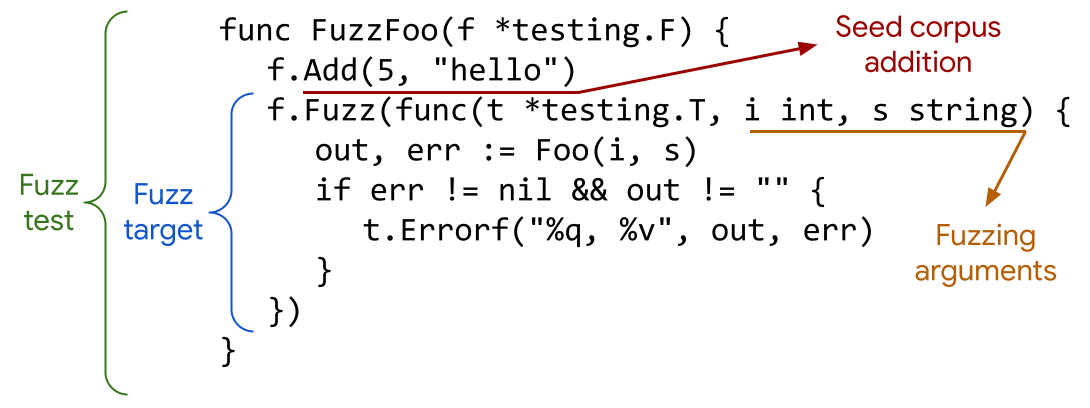# 史上最強程式碼自測方法，沒有之一！`go fuzzing` 至今已經發現了程式碼質量極高的 `Go` 標準庫超過200個bug，見：https://github.com/dvyukov/go-fuzz#trophies

Go 1.18 大家最關注的是泛型，然而我真的覺得 `go fuzzing` 真的是 Go 1.18 最有用的功能，沒有之一！

• 是什麼？
• 怎麼用？
• 有何最佳實踐？

Go 1.18 雖然還未正式釋出，但你可以下載 RC 版本，而且即使你生產用 Go 更早版本，你也可以開發環境使用 go fuzzing 尋找 bug

## go fuzzing 是什麼## go fuzzing 怎麼用

• 函式必須是 Fuzz開頭，唯一的引數是 `*testing.F`，沒有返回值

• Fuzz tests 必須在 `*_test.go` 的檔案裡

• 上圖中的 `fuzz target` 是個方法呼叫 `(*testing.F).Fuzz`，第一個引數是 `*testing.T`，然後就是稱之為 `fuzzing arguments` 的引數，沒有返回值

• 每個 `fuzz test` 裡只能有一個 `fuzz target`

• 呼叫 `f.Add(…)` 的時候需要引數型別跟 `fuzzing arguments` 順序和型別都一致

• `fuzzing arguments` 只支援以下型別：

• `string`, `[]byte`
• `int`, `int8`, `int16`, `int32`/`rune`, `int64`
• `uint`, `uint8`/`byte`, `uint16`, `uint32`, `uint64`
• `float32`, `float64`
• `bool`
• `fuzz target` 不要依賴全域性狀態，會並行跑。

### 執行 `fuzzing tests`

``````// 具體程式碼見 https://github.com/zeromicro/go-zero/blob/master/core/mr/mapreduce_fuzz_test.go
func FuzzMapReduce(f *testing.F) {
...
}
``````

``````go test -fuzz=MapReduce
``````

``````fuzz: elapsed: 0s, gathering baseline coverage: 0/2 completed
fuzz: elapsed: 0s, gathering baseline coverage: 2/2 completed, now fuzzing with 10 workers
fuzz: elapsed: 3s, execs: 3338 (1112/sec), new interesting: 56 (total: 57)
fuzz: elapsed: 6s, execs: 6770 (1144/sec), new interesting: 62 (total: 63)
fuzz: elapsed: 9s, execs: 10157 (1129/sec), new interesting: 69 (total: 70)
fuzz: elapsed: 12s, execs: 13586 (1143/sec), new interesting: 72 (total: 73)
^Cfuzz: elapsed: 13s, execs: 14031 (1084/sec), new interesting: 72 (total: 73)
PASS
ok    github.com/zeromicro/go-zero/core/mr  13.169s
``````

## go-zero 的最佳實踐

1. 定義 `fuzzing arguments`，首先要想明白怎麼定義 `fuzzing arguments`，並通過給定的 `fuzzing arguments``fuzzing target`
2. 思考 `fuzzing target` 怎麼寫，這裡的重點是怎麼驗證結果的正確性，因為 `fuzzing arguments` 是“隨機”給的，所以要有個通用的結果驗證方法
3. 思考遇到失敗的 case 如何列印結果，便於生成新的 `unit test`
4. 根據失敗的 `fuzzing test` 列印結果編寫新的 `unit test，這個新的 `unit test`會被用來除錯解決`fuzzing test`發現的問題，並固化下來留給`CI` 用`

``````func Sum(vals []int64) int64 {
var total int64

for _, val := range vals {
if val%1e5 != 0 {
total += val
}
}

}
``````

### 1. 定義 `fuzzing arguments`

``````func FuzzSum(f *testing.F) {
f.Fuzz(func(t *testing.T, n int) {
n %= 20
...
})
}
``````

### 2. 怎麼寫 `fuzzing target`

``````func FuzzSum(f *testing.F) {
rand.Seed(time.Now().UnixNano())

f.Fuzz(func(t *testing.T, n int) {
n %= 20
var vals []int64
var expect int64
for i := 0; i < n; i++ {
val := rand.Int63() % 1e6
vals = append(vals, val)
expect += val
}

assert.Equal(t, expect, Sum(vals))
})
}
``````

``````\$ go test -fuzz=Sum
fuzz: elapsed: 0s, gathering baseline coverage: 0/2 completed
fuzz: elapsed: 0s, gathering baseline coverage: 2/2 completed, now fuzzing with 10 workers
fuzz: elapsed: 0s, execs: 6672 (33646/sec), new interesting: 7 (total: 6)
--- FAIL: FuzzSum (0.21s)
--- FAIL: FuzzSum (0.00s)
sum_fuzz_test.go:34:
Error Trace:  sum_fuzz_test.go:34
value.go:556
value.go:339
fuzz.go:334
Error:        Not equal:
expected: 8736932
actual  : 8636932
Test:         FuzzSum

Failing input written to testdata/fuzz/FuzzSum/739002313aceff0ff5ef993030bbde9115541cabee2554e6c9f3faaf581f2004
To re-run:
go test -run=FuzzSum/739002313aceff0ff5ef993030bbde9115541cabee2554e6c9f3faaf581f2004
FAIL
exit status 1
FAIL  github.com/kevwan/fuzzing  0.614s
``````

### 3. 失敗 case 如何列印輸入

``````func FuzzSum(f *testing.F) {
rand.Seed(time.Now().UnixNano())

f.Fuzz(func(t *testing.T, n int) {
n %= 20
var vals []int64
var expect int64
var buf strings.Builder
buf.WriteString("\n")
for i := 0; i < n; i++ {
val := rand.Int63() % 1e6
vals = append(vals, val)
expect += val
buf.WriteString(fmt.Sprintf("%d,\n", val))
}

assert.Equal(t, expect, Sum(vals), buf.String())
})
}
``````

``````\$ go test -fuzz=Sum
fuzz: elapsed: 0s, gathering baseline coverage: 0/2 completed
fuzz: elapsed: 0s, gathering baseline coverage: 2/2 completed, now fuzzing with 10 workers
fuzz: elapsed: 0s, execs: 1402 (10028/sec), new interesting: 10 (total: 8)
--- FAIL: FuzzSum (0.16s)
--- FAIL: FuzzSum (0.00s)
sum_fuzz_test.go:34:
Error Trace:  sum_fuzz_test.go:34
value.go:556
value.go:339
fuzz.go:334
Error:        Not equal:
expected: 5823336
actual  : 5623336
Test:         FuzzSum
Messages:
799023,
110387,
811082,
115543,
859422,
997646,
200000,
399008,
7905,
931332,
591988,

To re-run:
FAIL
exit status 1
FAIL  github.com/kevwan/fuzzing  0.602s
``````

### 4. 編寫新的測試用例

``````func TestSumFuzzCase1(t *testing.T) {
vals := []int64{
799023,
110387,
811082,
115543,
859422,
997646,
200000,
399008,
7905,
931332,
591988,
}
assert.Equal(t, int64(5823336), Sum(vals))
}
``````

## `go fuzzing` 更多經驗

### Go 版本問題

``````//go:build go1.18
// +build go1.18

``````

### go fuzzing 不能復現的失敗

``````go test -fuzz=MapReduce
fuzz: elapsed: 0s, gathering baseline coverage: 0/2 completed
fuzz: elapsed: 0s, gathering baseline coverage: 2/2 completed, now fuzzing with 10 workers
fuzz: elapsed: 3s, execs: 3681 (1227/sec), new interesting: 54 (total: 55)
...
fuzz: elapsed: 1m21s, execs: 92705 (1101/sec), new interesting: 85 (total: 86)
--- FAIL: FuzzMapReduce (80.96s)
fuzzing process hung or terminated unexpectedly: exit status 2
To re-run:
FAIL
exit status 1
FAIL  github.com/zeromicro/go-zero/core/mr  81.471s
``````

``````func TestSumFuzzRandom(t *testing.T) {
const times = 100000
rand.Seed(time.Now().UnixNano())

for i := 0; i < times; i++ {
n := rand.Intn(20)
var vals []int64
var expect int64
var buf strings.Builder
buf.WriteString("\n")
for i := 0; i < n; i++ {
val := rand.Int63() % 1e6
vals = append(vals, val)
expect += val
buf.WriteString(fmt.Sprintf("%d,\n", val))
}

assert.Equal(t, expect, Sum(vals), buf.String())
}
}
``````

``````//go:build fuzz
// +build fuzz

``````

``````go test -tags fuzz ./...
``````

## 專案地址

https://github.com/zeromicro/go-zero

https://gitee.com/kevwan/go-zero